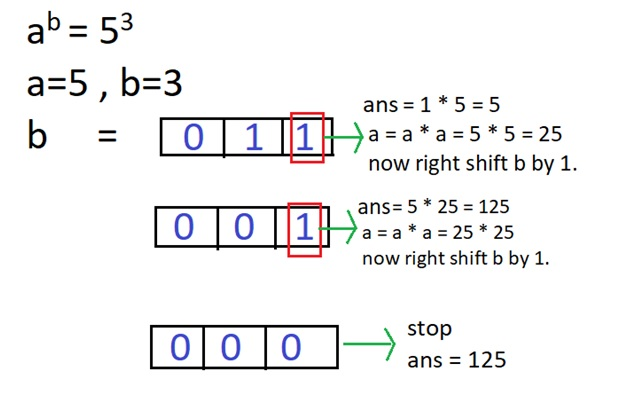Here, we are going to learn about the Fast Exponentiation using Bitmasking to compute the a raise to the power b i.e. (a^b).
Submitted by Vivek Kothari, on December 15, 2018

Problem statement: We have given two numbers "a" and "b". Now compute the value of "a" raise to the power "b" i.e. (a^b) by using bitmasks.

Example:

```    Input:
a = 3 , b = 5

Output:
a^b = 243
```

Algorithm:

This method uses bitmasks and without using extra space to calculate a^b in O(log b) time.

1. Let's take variable ans in which we store the result and initialize it with 1.
2. Extract last bit from b.
3. If the Extracted bit is 1 multiply ans with a, otherwise if the Extracted bit is 0 then don't multiply.
4. Update a to a*a.
5. Discard rightmost bit i.e right shift b by 1.
6. Repeat steps 2 to 5 until b becomes 0.
```    ans = 1
while(b>0)
{
if(b&1){
ans = (ans * a);
}
a = a*a;
b = b>>1;
}
//Now ans contains the value of a^b.
```

Explanation with example:## C++ program to implement Fast Exponentiation using Bitmasking

```#include<bits/stdc++.h>
using namespace std;

long long calculate(int a,int b)
{
// initialize ans with 1
long long ans = 1;
while(b>0)
{
// check if last bit 1
if(b&1){
ans = (ans * a);
}

// update value of a by a*a
a = a*a;

// right shift b by 1
b = b>>1;
}

return ans;
}

int main()
{
int a,b;
long long ans;
cout<<"enter the value of a and b : ";
cin>>a>>b;

cout<<"a^b = "<<calculate(a,b)<<endl<<endl;
return 0;
}
```

Output

```First run:
enter the value of a and b : 5 3
a^b = 125

Second run:
enter the value of a and b : 37 7
a^b = 94931877133

Third run:
enter the value of a and b : 37 0
a^b = 1
```

What's New

Top Interview Coding Problems/Challenges!

Languages: » C » C++ » C++ STL » Java » Data Structure » C#.Net » Android » Kotlin » SQL
Web Technologies: » PHP » Python » JavaScript » CSS » Ajax » Node.js » Web programming/HTML
Solved programs: » C » C++ » DS » Java » C#
Aptitude que. & ans.: » C » C++ » Java » DBMS
Interview que. & ans.: » C » Embedded C » Java » SEO » HR
CS Subjects: » CS Basics » O.S. » Networks » DBMS » Embedded Systems » Cloud Computing
» Machine learning » CS Organizations » Linux » DOS
More: » Articles » Puzzles » News/Updates